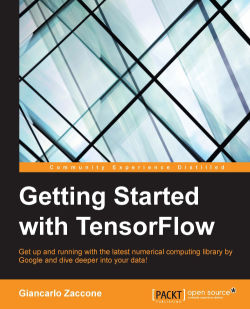### Getting Started with TensorFlow4.6 (11 reviews total)
By Giancarlo Zaccone
• Constantly updated with 100+ new titles each month
• Breadth and depth in over 1,000+ technologies

Google's TensorFlow engine, after much fanfare, has evolved in to a robust, user-friendly, and customizable, application-grade software library of machine learning (ML) code for numerical computation and neural networks.

This book takes you through the practical software implementation of various machine learning techniques with TensorFlow. In the first few chapters, you'll gain familiarity with the framework and perform the mathematical operations required for data analysis. As you progress further, you'll learn to implement various machine learning techniques such as classification, clustering, neural networks, and deep learning through practical examples.

By the end of this book, you’ll have gained hands-on experience of using TensorFlow and building classification, image recognition systems, language processing, and information retrieving systems for your application.

Publication date:
July 2016
Publisher
Packt
Pages
180
ISBN
9781786468574

## ChapterÂ 1.Â TensorFlow â€“ Basic Concepts

In this chapter, we'll cover the following topics:

• Machine learning and deep learning basics

• TensorFlowÂ â€“ A general overview

• Python basics

• Installing TensorFlow

• First working session

• Data Flow Graph

• TensorFlow programming model

• How to use TensorBoard

## Machine learning and deep learning basics

Machine learning is a branch of artificial intelligence, and more specifically of computer science, which deals with the study of systems and algorithms that can learn from data, synthesizing new knowledge from them.

The word learn intuitively suggests that a system based on machine learning, may, on the basis of the observation of previously processed data, improve its knowledge in order to achieve better results in the future, or provide output closer to the desired output for that particular system.

The ability of a program or a system based on machine learning to improve its performance in a particular task, thanks to past experience, is strongly linked to its ability to recognize patterns in the data. This theme, called pattern recognition, is therefore of vital importance and of increasing interest in the context of artificial intelligence; it is the basis of all machine learning techniques.

The training of a machine learning system can be done in different ways:

• Supervised learning

• Unsupervised learning

### Supervised learning

Supervised learning is the most common form of machine learning. With supervised learning, a set of examples, the training set, is submitted as input to the system during the training phase, where each example is labeled with the respective desired output value. For example, let's consider a classification problem, where the system must attribute some experimental observations in one of the N different classes already known. In this problem, the training set is presented as a sequence of pairs of the type `{(X1, Y1), ....., (Xn, Yn)}` where `Xi` are the input vectors (feature vectors) and `Yi` represents the desired class for the corresponding input vector. Most supervised learning algorithms share one characteristic: the training is performed by the minimization of a particular loss function (cost function), which represents the output error with respect to the desired output system.

The cost function most used for this type of training calculates the standard deviation between the desired output and the one supplied by the system. After training, the accuracy of the model is measured on a set of disjointed examples from the training set, the so-called validation set.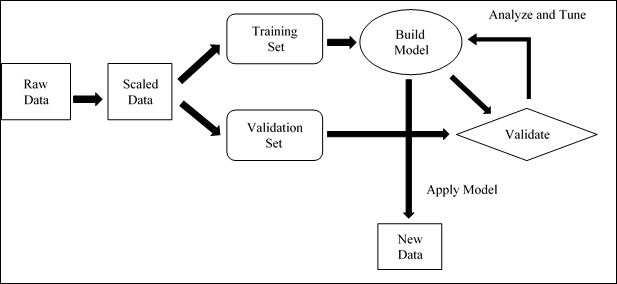Supervised learning workflow

In this phase the model's generalization capability is then verified: we will test if the output is correct for an unused input during the training phase.

#### Unsupervised learning

In unsupervised learning, the training examples provided by the system are not labeled with the related belonging class. The system, therefore, develops and organizes the data, looking for common characteristics among them, and changing them based on their internal knowledge.

Unsupervised learning algorithms are particularly used in clustering problems, in which a number of input examples are present, you do not know the class a priori, and you do not even know what the possible classes are, or how numerous they are. This is a clear case when you cannot use supervised learning, because you do not know a priori the number of classes.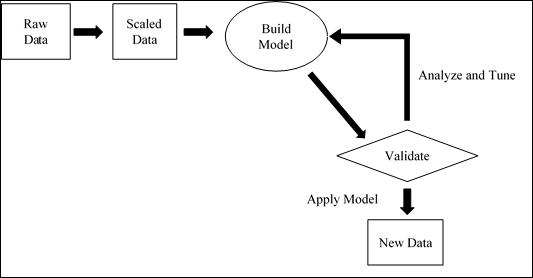Unsupervised learning workflow

#### Deep learning

Deep learning techniques represent a remarkable step forward taken by machine learning in recent decades, having provided results never seen before in many applications, such as image and speech recognition or Natural Language Processing (NLP). There are several reasons that led to deep learning being developed and placed at the center of the field of machine learning only in recent decades. One reason, perhaps the main one, is surely represented by progress in hardware, with the availability of new processors, such as graphics processing units (GPUs), which have greatly reduced the time needed for training networks, lowering them by a factor of 10 or 20. Another reason is certainly the ever more numerous datasets on which to train a system, needed to train architectures of a certain depth and with a high dimensionality for the input data.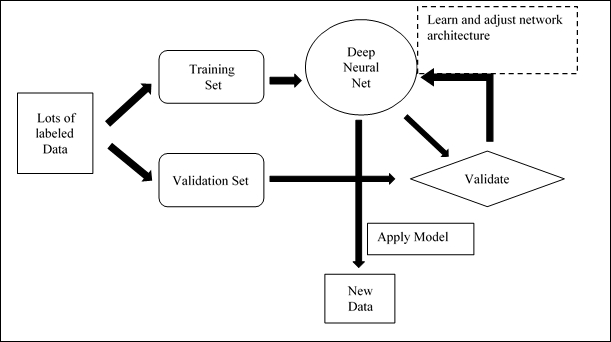Deep learning workflow

Deep learning is based on the way the human brain processes information and learns, responding to external stimuli. It consists in a machine learning model at several levels of representation in which the deeper levels take as input the outputs of the previous levels, transforming them and always abstracting more. Each level corresponds in this hypothetical model to a different area of the cerebral cortex: when the brain receives images, it processes them through various stages such as edge detectionÂ andÂ form perception, that is, from a primitive representation level to the most complex. For example, in an image classification problem, each block gradually extracts the features, at various levels of abstraction, inputting of data already processed, by means of filtering operations.

## TensorFlow â€“ A general overview

TensorFlow (https://www.tensorflow.org/) is a software library, developed by Google Brain Team within Google's Machine Learning Intelligence research organization, for the purposes of conducting machine learning and deep neural network research. TensorFlow then combines the computational algebra of compilation optimization techniques, making easy the calculation of many mathematical expressions where the problem is the time required to perform the computation.

The main features include:

• Defining, optimizing, and efficiently calculating mathematical expressions involving multi-dimensional arrays (tensors).

• Programming support of deep neural networks and machine learning techniques.

• Transparent use of GPU computing, automating management and optimization of the same memory and the data used. You can write the same code and run it either on CPUs or GPUs. More specifically, TensorFlow will figure out which parts of the computation should be moved to the GPU.

• High scalability of computation across machines and huge data sets.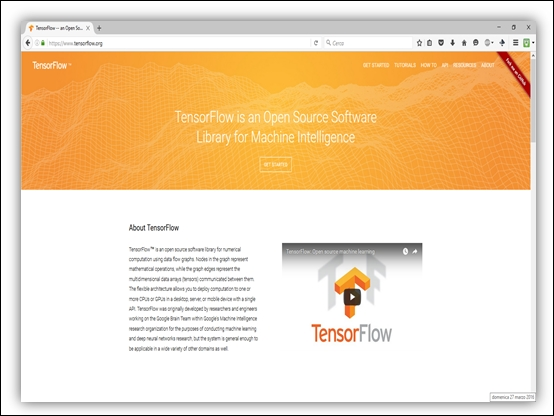TensorFlow is available with Python and C++ support, and we shall use Python 2.7 for learning, as indeed Python API is better supported and much easier to learn. The Python installation depends on your systems; the download page (https://www.python.org/downloads/) contains all the information needed for its installation. In the next section, we explain very briefly the main features of the Python language, with some programming examples.

## Python basics

Python is a strongly typed and dynamic language (data types are necessary but it is not necessary to explicitly declare them), case-sensitive (var and VAR are two different variables), and object-oriented (everything in Python is an object).

### Syntax

In Python, a line terminator is not required, and the blocks are specified with the indentation. Indent to begin a block and remove indentation to conclude it, that's all. Instructions that require an indented block end with a colon (`:`). Comments begin with the hash sign (`#`) and are single-line. Strings on multiple lines are used for multi-line comments. Assignments are accomplished with the equal sign (`=`). For equality tests we use the double equal (`==`) symbol. You can increase and decrease a value by using `+=` and `-=` followed by the addend. This works with many data types, including strings. You can assign and use multiple variables on the same line.

Following are some examples:

```>>> myvar = 3
>>> myvar += 2
>>> myvar
5
>>> myvar -= 1
>>> myvar
4
"""This is a comment"""
>>> mystring = "Hello"
>>> mystring += " world."
>>> print mystring
Hello world.
```

The following code swaps two variables in one line:

```>>> myvar, mystring = mystring, myvar
```

### Data types

The most significant structures in Python are lists, tuples, and dictionaries. The sets are integrated in Python since version 2.5 (for previous versions, they are available in the sets library). Lists are similar to single-dimensional arrays but you can create lists that contain other lists. Dictionaries are arrays that contain pairs of keys and values (hash table), and tuples are immutable mono-dimensional objects. In Python arrays can be of any type, so you can mix integers, strings, and so on in your lists/dictionaries and tuples. The index of the first object in any type of array is always zero. Negative indices are allowed and counting from the end of the array, `-1` is the last element. Variables can refer to functions.

```>>> example = [1, ["list1", "list2"], ("one", "tuple")]
>>> mylist = ["Element 1", 2, 3.14]
>>> mylist 
"Element 1"
>>> mylist [-1]
3.14
>>> mydict = {"Key 1": "Val 1", 2: 3, "pi": 3.14}
>>> mydict ["pi"]
3.14
>>> mytuple = (1, 2, 3)
>>> myfunc = len
>>> print myfunc (mylist)
3
```

You can get an array range using a colon (`:`). Not specifying the starting index of the range implies the first element; not indicating the final index implies the last element. Negative indices count from the last element (`-1` is the last element). Then run the following command:

```>>> mylist = ["first element", 2, 3.14]
>>> print mylist [:]
['first element', 2, 3.1400000000000001]
>>> print mylist [0:2]
['first element', 2]
>>> print mylist [-3:-1]
['first element', 2]
>>> print mylist [1:]
[2, 3.14]
```

### Strings

Python strings are indicated either with a single quotation mark (`'`) or double (`"`) and are allowed to use a notation within a delimited string on the other (`"He said' hello '."It is valid`). Strings of multiple lines are enclosed in triple (or single) quotes (`"""`). Python supports unicode; just use the syntax: `"This is a unicode string"`. To insert values into a string , use the `%` operator (modulo) and a tuple. Each `%` is replaced by a tuple element, from left to right, and is permitted to use a dictionary for the replacements.

```>>> print "Nome: %s\nNumber: %s\nString: %s" % (myclass.nome, 3, 3 * "-")
Name: Poromenos
Number: 3
String: ---
strString = """this is a string
on multiple lines."""
>>> print "This %(verbo)s un %(name)s." % {"name": "test", "verb": "is"}
This is a test.
```

### Control flow

The instructions for flow control are `if`, `for`, and `while`. There is the `select` control flow; in its place, we useÂ `if`. The `for` control flow is used to enumerate the members of a list. To get a list of numbers, you use `range (number)`.

```rangelist = range(10)
>>> print rangelist
[0, 1, 2, 3, 4, 5, 6, 7, 8, 9]
```

Let's check if `number` is one of the numbers in the tuple:

```for number in rangelist:
if number in (3, 4, 7, 9):
# "Break" ends the for instruction without the else clause
break
else:
# "Continue" continues with the next iteration of the loop
continue
else:
# this is an optional "else"
# executed only if the loop is not interrupted with "break".
pass # it does nothing
if rangelist == 2:
print "the second element (lists are 0-based) is 2"
elif rangelist == 3:
print "the second element is 3"
else:
print "I don't know"
while rangelist == 1:
pass
```

### Functions

Functions are declared with the keyword `def`. Any optional arguments must be declared after those that are mandatory and must have a value assigned. When calling functions using arguments to name you must also pass the value. Functions can return a tuple (tuple unpacking enables the return of multiple values). Lambda functions are in-line. Parameters are passed by reference, but immutable types (tuples, integers, strings, and so on) cannot be changed in the function. This happens because it is only passed through the position of the element in memory, and assigning another object to the variable results in the loss of the object reference earlier.

For example:

```# equal to a def f(x): return x + 1
funzionevar = lambda x: x + 1
>>> print funzionevar(1)
2
def passing_example(my_list,my_int):
my_list.append("new element")
my_int = 4
return my_list, my_int
>>> input_my_list = [1, 2, 3]
>>> input_my_int = 10
>>> print passing_example(input_my_list, input_my_int)
([1, 2, 3, 'new element'], 10)
>>> my_list
[1, 2, 3, 'new element']
>>> my_int
10
```

### Classes

Python supports multiple inheritance of classes. The variables and private methods are declared by convection (it is not a rule of language) by preceding them with two underscores (`__`). We can assign attributes (properties) to arbitrary instances of a class.

The following is an example:

```class Myclass:
common = 10
def __init__(self):
self.myvariable= 3
def myfunc(self, arg1, arg2):
return self.myvariable
# We create an instance of the class
>>> instance= Myclass()
>>> instance.myfunc(1, 2)
3
# This variable is shared by all instances
>>> instance2= Myclass()
>>> instance.common
10
>>> instance2.common
10
# Note here how we use the class name
>>> Myclass.common = 30
>>> instance.common
30
>>> instance2.common
30
# This does not update the variable in the class,
# Instead assign a new object to the variable
# of the first instance.
>>> instance.common = 10
>>> instance.common
10
>>> instance2.common
30
>>> Myclass.common = 50
# The value is not changed because "common" is an instance variable.
>>> instance.common
10
>>> instance2.common
50
# This class inherits from Myclass. Multiple inheritance
# is declared like this:
# class AltraClasse(Myclass1, Myclass2, MyclassN)
class AnotherClass(Myclass):
# The topic "self" is automatically passed
# and makes reference to instance of the class, so you can set
# of instance variables as above, but within the class.
def __init__(self, arg1):
self.myvariable= 3
print arg1
>>> instance= AnotherClass ("hello")
hello
>>> instance.myfunc(1, 2)
3
# This class does not have a member (property) .test member, but
# We can add one all instance when we want. Note
# .test That will be a member of only one instance.
>>> instance.test = 10
>>> instance.test
10
```

### Exceptions

Exceptions in Python are handled with` try-except` blocks [`exception_name`]:

```def my_func():
try:
# Division by zero causes an exception
10 / 0
except ZeroDivisionError:
print "Oops, error"
else:
# no exception, let's proceed
pass
finally:
# This code is executed when the block
# Try..except is already executed and all exceptions
# Were handled, even if there is a new
# Exception directly in the block.
print "finish"
>>> my_func()
Oops, error.
finish
```

### Importing a library

External libraries are imported with `import [library name]`. You can also use the form `[libraryname] import [funcname]` to import individual features. Here's an example:

```import random
from time import clock
randomint = random.randint(1, 100)
>>> print randomint
64
```

## Installing TensorFlow

The TensorFlow Python API supports Python 2.7 and Python 3.3+. The GPU version (Linux only) requires the Cuda Toolkit >= 7.0 and cuDNN >= v2.

When working in a Python environment, it is recommended you use `virtualenv`. It will isolate your Python configuration for different projects; using `virtualenv`Â  will not overwrite existing versions of Python packages required by TensorFlow.

### Installing on Mac or Linux distributions

The following are the steps to install TensorFlow on Mac and Linux system:

1. First install pip and virtualenv (optional) if they are not already installed:

Â  Â  Â  Â  Â For Ubuntu/Linux 64-bit:

```        \$ sudo apt-get install python-pip python-dev python-virtualenv
```

Â  Â  Â  Â  Â  Â  Â  Â For Mac OS X:

```        \$ sudo easy_install pip
\$ sudo pip install --upgrade virtualenv
```
2. Then you can create a virtual environment virtualenv. The following commands create a virtual environment virtualenv in the `~ / tensorflow` directory:

```    \$ virtualenv --system-site-packages ~/tensorflow
```
3. The next step is to activate virtualenv as follows:

```    \$ source ~/tensorflow/bin/activate.csh
(tensorflow)\$
```
4. Henceforth, the name of the environment we're working in precedes the command line. Once activated, Pip is used to install TensorFlow within it.

Â  Â  Â  Â  Â  Â  Â  Â For Ubuntu/Linux 64-bit, CPU:

```(tensorflow)\$ pip install --upgrade https://storage.googleapis.com/tensorflow/linux/cpu/tensorflow-0.5.0-cp27-none-linux_x86_64.whl
```

Â  Â  Â  Â  Â  Â  Â  Â  Â For Mac OS X, CPU:

```(tensorflow)\$ pip install --upgrade https://storage.googleapis.com/tensorflow/mac/tensorflow-0.5.0-py2-none-any.whl
```

If you want to use your GPU card with TensorFlow, then install another package. I recommend you visit the official documentation to see if your GPU meets the specifications required to support TensorFlow.

### Note

To enable your GPU with TensorFlow, you can refer to ( https://www.tensorflow.org/versions/r0.9/get_started/os_setup.html#optional-linux-enable-gpu-support) for a complete description.

Finally, when you've finished, you must disable the virtual environment:

```(tensorflow)\$ deactivate
```

### Installing on Windows

If you can't get a Linux-based system, you can install Ubuntu on a virtual machine; just use a free application called VirtualBox, which lets you create a virtual PC on Windows and install Ubuntu in the latter. So you can try the operating system without creating partitions or dealing with cumbersome procedures.

### Note

After installing VirtualBox, you can install Ubuntu ( www.ubuntu.com ) and then follow the installation for Linux machines to install TensorFlow.

### Installation from source

However, it may happen that the Pip installation causes problems, particularly when using the visualization tool TensorBoard (see https://github.com/tensorflow/tensorflow/issues/530 ). To fix this problem, I suggest you build and install TensorFlow, starting form source files, through the following steps:

1. Clone the TensorFlow repository:

```git clone --recurse-submodules

```

https://github.com/tensorflow/tensorflow

2. Install Bazel (dependencies and installer), following the instructions at:

3. Run the Bazel installer:

```   chmod +x bazel-version-installer-os.sh
./bazel-version-installer-os.sh --user
```
4. Install the Python dependencies:

```sudo apt-get install python-numpy swig python-dev
```

```./configure
```
6. Create your own TensorFlow Pip package using `bazel`:

```bazel build -c opt //tensorflow/tools/pip_package:build_pip_package
```
7. To build with GPU support, use `bazel build -c opt --config=cuda` followed again by:

```//tensorflow/tools/pip_package:build_pip_package
```
8. Finally, install TensorBoard where the name of the `.whl` file will depend on your platform.

```   pip install /tmp/tensorflow_pkg/tensorflow-0.7.1-py2-none- linux_x86_64.whl
```
9. Good Luck!

### Note

Please refer to https://www.tensorflow.org/versions/r0.7/get_started/os_setup.html#installation-for-linux for further information.

Open a terminal and type the following lines of code:

```>>> import tensorflow as tf
>>> hello = tf.constant("hello TensorFlow!")
>>> sess=tf.Session()
```

To verify your installation, just type:

```>>> print(sess.run(hello))
```

You should have the following output:

```Hello TensorFlow!
>>>
```

## First working session

Finally it is time to move from theory to practice. I will use the Python 2.7 IDE to write all the examples. To get an initial idea of how to use TensorFlow, open the Python editor and write the following lines of code:

```x = 1
y = x + 9
print(y)
import tensorflow as tf
x = tf.constant(1,name='x')
y = tf.Variable(x+9,name='y')
print(y)
```

As you can easily understand in the first three lines, the constant `x`, set equal to `1`, is then added to `9` to set the new value of the variable `y`, and then the end result of the variable `y` is printed on the screen.

In the last four lines, we have translated according to TensorFlow library the first three variables.

If we run the program, we have the following output:

```10
<tensorflow.python.ops.variables.Variable object at    0x7f30ccbf9190>
```

The TensorFlow translation of the first three lines of the program example produces a different result. Let's analyze them:

1. The following statement should never be missed if you want to use the TensorFlow library. It tells us that we are importing the library and call it `tf`:

```import tensorflow as tf
```
2. We create a constant value called `x`, with a value equal to one:

```x = tf.constant(1,name='x')
```
3. Then we create a variable called `y`. This variable is defined with the simple equation `y=x+9`:

```y = tf.Variable(x+9,name='y')
```
4. Finally, print out the result:

```print(y)
```

So how do we explain the different result? The difference lies in the variable definition. In fact, the variable `y` doesn't represent the current value of `x + 9`, instead it means: when the variable y is computed, take the value of the constant x and add 9 to it. This is the reason why the value of `y` has never been carried out. In the next section, I'll try to fix it.

So we open the Python IDE and enter the following lines: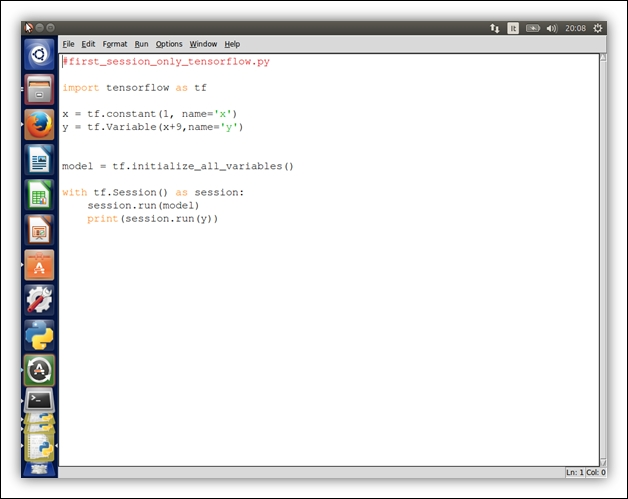Running the preceding code, the output result is finally as follows:

```10
```

We have removed the print instruction, but we have initialized the model variables:

```model = tf.initialize_all_variables()
```

And, mostly, we have created a session for computing values. In the next step, we run the model, created previously, and finally run just the variable `y` and print out its current value.

```with tf.Session() as session:
session.run(model)
print(session.run(y))
```

This is the magic trick that permits the correct result. In this fundamental step, the execution graph called Data Flow Graph is created in the `session`, with all the dependencies between the variables. The `y` variable depends on the variable `x`, and that value is transformed by adding `9` to it. The value is not computed until the session is executed.

This last example introduced another important feature in TensorFlow, the Data Flow Graph.

## Data Flow Graphs

A machine learning application is the result of the repeated computation of complex mathematical expressions. In TensorFlow, a computation is described using the Data Flow Graph, where each node in the graph represents the instance of a mathematical operation (`multiply`, `add`, `divide`, and so on), and each edge is a multi-dimensional data set (tensors) on which the operations are performed.

TensorFlow supports these constructs and these operators. Let's see in detail how nodes and edges are managed by TensorFlow:

• Node: In TensorFlow, each node represents the instantion of an operation. Each operation has `>=` inputs and `>= 0` outputs.

• Edges: In TensorFlow, there are two types of edge:

• Normal Edges: They are carriers of data structures (tensors), where an output of one operation (from one node) becomes the input for another operation.

• Special Edges: These edges are not data carriers between the output of a node (operator) and the input of another node. A special edge indicates a control dependency between two nodes. Let's suppose we have two nodes A and B and a special edges connecting A to B; it means that B will start its operation only when the operation in A ends. Special edges are used in Data Flow Graph to set the happens-before relationship between operations on the tensors.

Let's explore some features in Data Flow GraphÂ in greater detail:

• Operation: This represents an abstract computation, such as adding or multiplying matrices. An operation manages tensors. It can just be polymorphic: the same operation can manipulate different tensor element types. For example, the addition of two int32 tensors, the addition of two float tensors, and so on.

• Kernel: This represents the concrete implementation of that operation. A kernel defines the implementation of the operation on a particular device. For example, an add matrix operation can have a CPU implementation and a GPU one. In the following section, we have introduced the concept of sessions to create a `del` execution graph in TensorFlow. Let's explain this topic:

• Session: When the client program has to establish communication with the TensorFlow runtime system, a session must be created. As soon as the session is created for a client, an initial graph is created and is empty. It has two fundamental methods:

• `session.extend`: In a computation, the user can extend the execution graph, requesting to add more operations (nodes) and edges (data).

• `session.run`: Using TensorFlow, sessions are created with some graphs, and these full graphs are executed to get some outputs, or sometimes, subgraphs are executed thousands/millions of times using run invocations. Basically, the method runs the execution graph to provide outputs.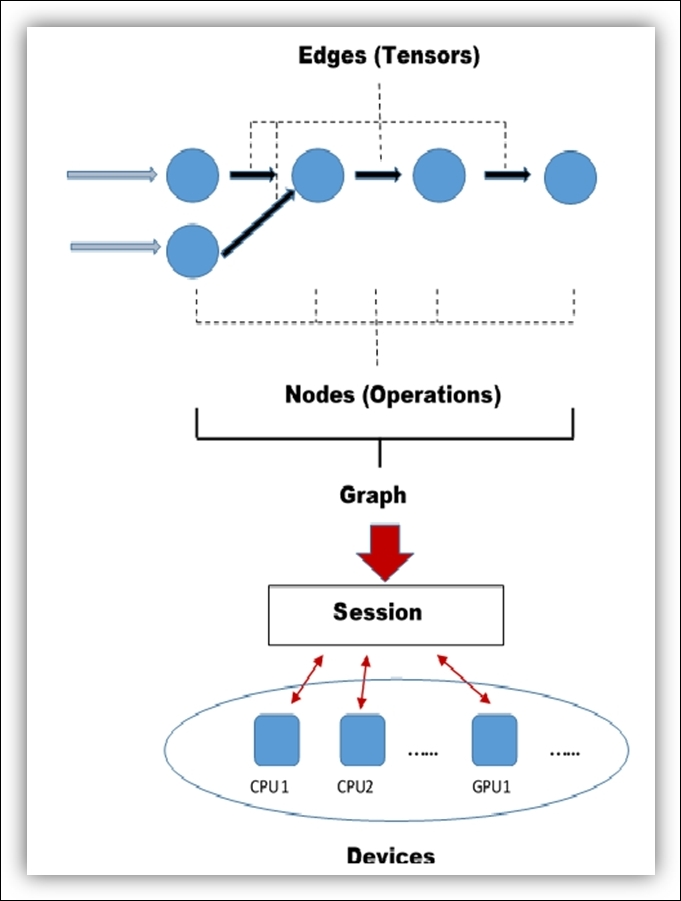Features in Data Flow Graph

## TensorFlow programming model

Adopting Data Flow Graph as execution model, you divide the data flow design (graph building and data flow) from its execution (CPU, GPU cards, or a combination), using a single programming interface that hides all the complexities. It also defines what the programming model should be like in TensorFlow.

Let's consider the simple problem of multiplying two integers, namely `a` and `b`.

The following are the steps required for this simple problem:

1. Define and initialize the variables. Each variable should define the state of a current execution. After importing the TensorFlow module in Python:

```import tensorflow as tf
```
2. We define the variables `a` and `b` involved in the computation. These are defined via a more basic structure, called the `placeholder`:

```a = tf.placeholder("int32")
b = tf.placeholder("int32")
```
3. A `placeholder` allows us to create our operations and to build our computation graph, without needing the data.

4. Then we use these variables, as inputs for TensorFlow's function `mul`:

```y = tf.mul(a,b)
this function will return the result of the multiplication the input   integers a and b.
```
5. Manage the execution flow, this means that we must build a session:

```sess = tf.Session()
```
6. Visualize the results. We run our model on the variables `a` and `b`, feeding data into the data flow graph through the placeholders previously defined.

```print sess.run(y , feed_dict={a: 2, b: 5})
```

### How to use TensorBoard

TensorBoard is a visualization tool, devoted to analyzing Data Flow Graph and also to better understand the machine learning models. It can view different types of statistics about the parameters and details of any part of a computer graph graphically. It often happens that a graph of computation can be very complex. A deep neural network can have up to 36,000 nodes. For this reason, TensorBoard collapses nodes in high-level blocks, highlighting the groups with identical structures. Doing so allows a better analysis of the graph, focusing only on the core sections of the computation graph. Also, the visualization process is interactive; user can pan, zoom, and expand the nodes to display the details.

The following figure shows a neural network model with TensorBoard: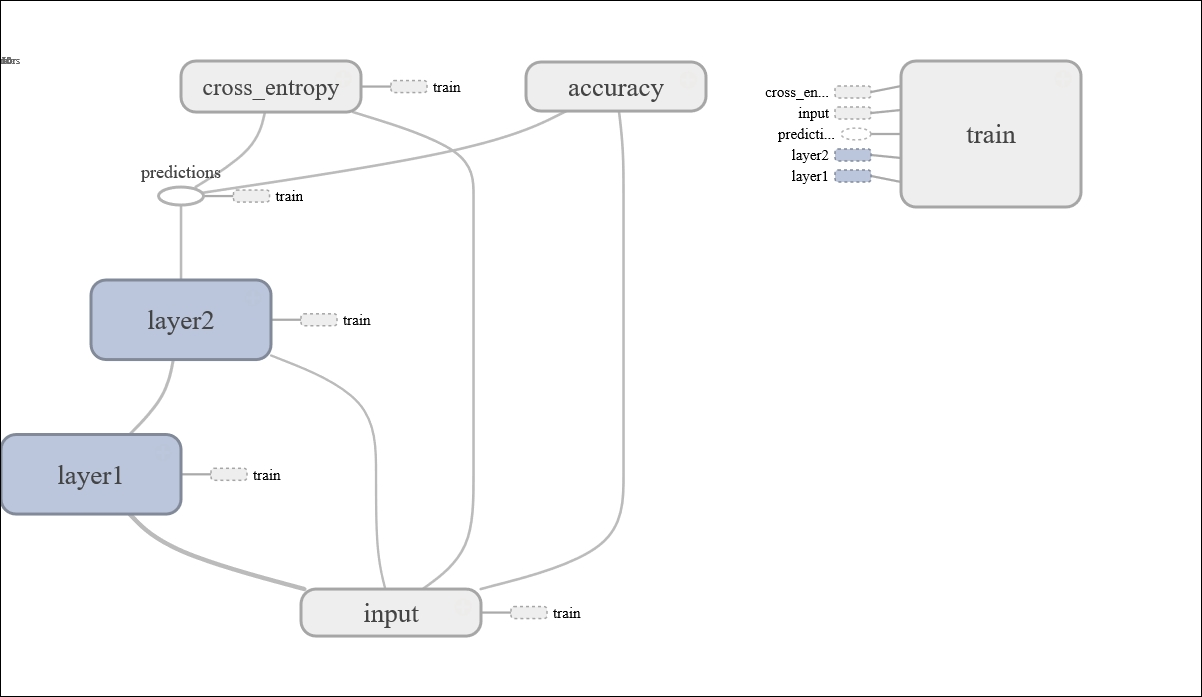A TensorBoard visualization example

TensorBoard's algorithms collapse nodes into high-level blocks and highlight groups with the same structures, while also separating out high-degree nodes. The visualization tool is also interactive: the users can pan, zoom in, expand, and collapse the nodes.

TensorBoard is equally useful in the development and tuning of a machine learning model. For this reason, TensorFlow lets you insert so-called summary operations into the graph. These summary operations monitor changing values (during the execution of a computation) written in a log file. Then TensorBoard is configured to watch this log file with summary information and display how this information changes over time.

Let's consider a basic example to understand the usage of TensorBoard. We have the following example:

```import tensorflow as tf
a = tf.constant(10,name="a")
b = tf.constant(90,name="b")
y = tf.Variable(a+b*2, name="y")
model = tf.initialize_all_variables()
with tf.Session() as session:
merged = tf.merge_all_summaries()
writer = tf.train.SummaryWriter\
("/tmp/tensorflowlogs",session.graph)
session.run(model)
print(session.run(y))
```

That gives the following result:

```190
```

Let's point into the session management. The first instruction to consider is as follows:

```merged = tf.merge_all_summaries()
```

This instruction must merge all the summaries collected in the default graph.

Then we create `SummaryWriter`. It will write all the summaries (in this case the execution graph) obtained from the code's execution into the `/tmp/tensorflowlogs` directory:

```writer = tf.train.SummaryWriter\
("/tmp/tensorflowlogs",session.graph)
```

Finally, we run the model and so build the Data Flow Graph:

```session.run(model)
print(session.run(y))
```

The use of TensorBoard is very simple. Let's open a terminal and enter the following:

```\$tensorboard --logdir=/tmp/tensorflowlogs
```

A message such as the following should appear:

```startig tensorboard on port 6006
```

Then, by opening a web browser, we should display the Data Flow Graph with auxiliary nodes: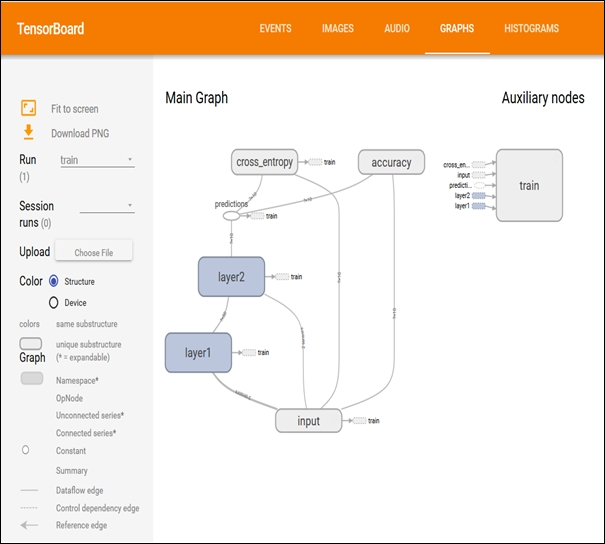Data Flow Graph display with TensorBoard

Now we will be able to explore the Data Flow Graph: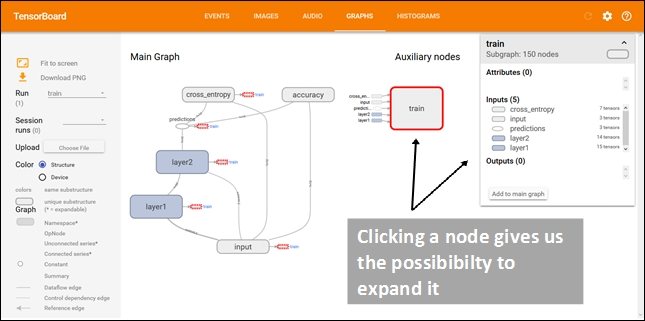Explore the Data Flow Graph display with TensorBoard

TensorBoard uses special icons for constants and summary nodes. To summarize, we report in the next figure the table of node symbols displayed: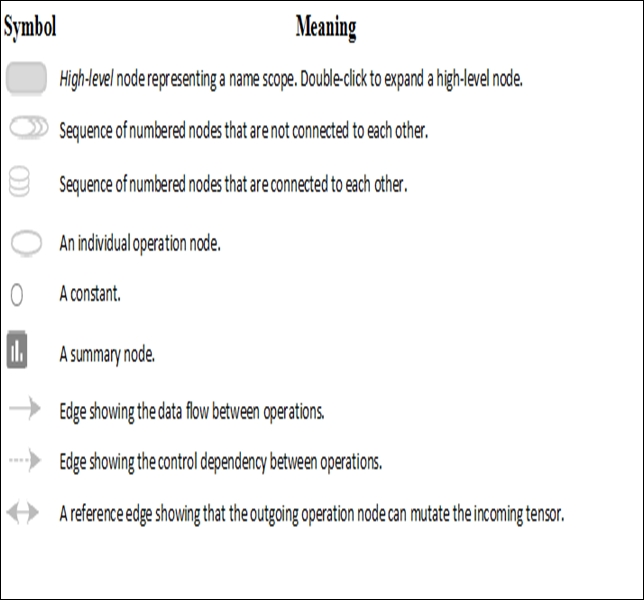Node symbols in TensorBoard

## Summary

In this chapter, we introduced the main topics:Â machine learning and deep learning. While machine learning explores the study and construction of algorithms that can learn from, and make predictions on data, deep learning is based precisely on the way the human brain processes information and learns, responding to external stimuli.

In this vast scientific research and practical application area, we can firmly place the TensorFlow software library, developed by the Google's research group for artificial intelligence (Google Brain Project) and released as open source software on November 9, 2015.

After electing the Python programming language as the development tool for our examples and applications, we saw how to install and compile the library, and then carried out a first working session. This allowed us to introduce the execution model of TensorFlow and Data Flow Graph. It led us to define what our programming model should be.

The chapter ended with an example of how to use an important tool for debugging machine learning applications: TensorBoard.

In the next chapter, we will continue our journey into the TensorFlow library, with the intention of showing its versatility. Starting from the fundamental concept, tensors, we will see how to use the library for purely math applications.